Win up to 100% scholarship on Aakash BYJU'S JEE/NEET courses with ABNAT Win up to 100% scholarship on Aakash BYJU'S JEE/NEET courses with ABNAT

# JEE Main 2022 June 26 – Shift 1 Chemistry Question Paper with Solutions

Candidates can get the JEE Main 2022 June 26 Shift 1 Chemistry Question Paper and Solutions here on this page, and verify their answers. Our subject experts have created these solutions to help you understand the method of solving them effortlessly. Solving the JEE main question papers will help you understand the exam pattern clearly and evaluate the difficulty level. This will further help you to plan and prepare for the upcoming JEE exams. You can also access JEE main answer keys for chemistry question papers. Below are the solutions for the June 26 Shift 1 JEE main 2022 chemistry question paper.
JEE Main 2022 June 26 – Shift 1 Chemistry Question Paper with Solutions

SECTION – A

Multiple Choice Questions: This section contains 20 multiple choice questions. Each question has 4 choices (1), (2), (3) and (4), out of which ONLY ONE is correct.

1. A commercially sold conc. HCl is 35% HCl by mass. If the density of this commercial acid is 1.46 g/mL, the molarity of this solution is :

(Atomic mass : Cl = 35.5 amu, H = 1 amu)

(A) 10.2 M

(B) 12.5 M

(C) 14.0 M

(D) 18.2 M

Sol. Molarity

$$\begin{array}{l}=\frac{35}{\left ( 36.5 \right )\times\frac{100}{1.46}}\times 1000=14.0~M\end{array}$$

2. An evacuated glass vessel weighs 40.0 g when empty, 135.0 g when filled with a liquid of density 0.95 g mL–1 and 40.5 g when filled with an ideal gas at 0.82 atm at 250 K. The molar mass of the gas in g mol–1 is:

(Given : R = 0.082 L atm K–1 mol–1)

(A) 35

(B) .50

(C) 75

(D) 175

Sol. Weight of liquid = 135.0 – 40.0 = 95.0 g

Volume of liquid

$$\begin{array}{l}=\frac{95}{0.95}=100~ml~=0.1L\end{array}$$

Weight of gas = 0.5 g

Moles of gas

$$\begin{array}{l}=\frac{0.5}{M}\end{array}$$

PV = nRT

$$\begin{array}{l}0.82\times0.1=\frac{0.5}{M}\times0.082\times250\end{array}$$
$$\begin{array}{l}M=0.5\times250=125~g~\textup{mol}^{-1}\end{array}$$

3. If the radius of the 3rd Bohr’s orbit of hydrogen atom is r3 and the radius of 4th Bohr’s orbit is r4. Then :

(A)

$$\begin{array}{l}r_4=\frac{9}{16}r_3\end{array}$$

(B)

$$\begin{array}{l}r_4=\frac{16}{9}r_3\end{array}$$

(C)

$$\begin{array}{l}r_4=\frac{3}{4}r_3\end{array}$$

(D)

$$\begin{array}{l}r_4=\frac{4}{3}r_3\end{array}$$

Sol.

$$\begin{array}{l}r_n=r_0\frac{n^2}{Z}\end{array}$$
$$\begin{array}{l}r_4=r_0\times\frac{4^2}{1}\end{array}$$

and

$$\begin{array}{l}r_3=r_0\times\frac{3^2}{1}\end{array}$$

Therefore,

$$\begin{array}{l}\frac{r_4}{r_3}=\frac{4^2}{3^2}\Rightarrow r_4=\frac{16}{9}r_3\end{array}$$

4. Consider the ions/molecules

$$\begin{array}{l}O_2^+,O_2,O_2^-,O_2^{2-}\end{array}$$

For increasing bond order the correct option is:

(A)

$$\begin{array}{l}O_{2}^{2-}<O_2^-<O_2<O_2^+\end{array}$$

(B)

$$\begin{array}{l}O_{2}^-<O_2^{2-}<O_2<O_2^+\end{array}$$

(C)

$$\begin{array}{l}O_{2}^-<O_2^{2-}<O_2^+<O_2\end{array}$$

(D)

$$\begin{array}{l}O_{2}^-<O_2^+<O_2^{2-}<O_2\end{array}$$

Sol.

Species

Bond order

$$\begin{array}{l}O_2^+\end{array}$$

2.5

O2

2

$$\begin{array}{l}O_2^-\end{array}$$

1.5

$$\begin{array}{l}O_2^{-2}\end{array}$$

1

5. The [

$$\begin{array}{l}\left ( \frac{\partial E}{\partial T}\right )_P\end{array}$$
of different types of half cells are as follows:

A

B

C

D

1 × 10-4

2 × 10-4

0.1 × 10-4

0.2 × 10-4

(Where E is the electromotive force)

Which of the above half cells would be preferred to be used as reference electrode?

(A) A

(B) B

(C) C

(D) D

Sol. We know that

$$\begin{array}{l}E=E^\circ-\frac{2.303RT}{nF}log\left [ \frac{1}{\textup{oxidized~form}} \right ]\end{array}$$
$$\begin{array}{l}\textup{Slope}=\frac{-2.303R}{nF}log\left [ \frac{1}{\textup{oxidized~form}} \right ]\end{array}$$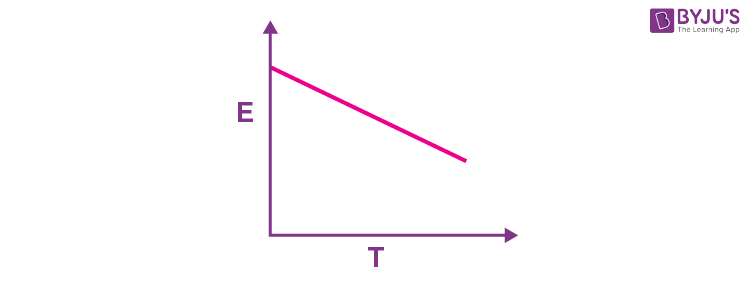Higher the value of slope

$$\begin{array}{l}\left ( \frac{\partial E}{\partial T} \right )_P\end{array}$$
more the deviation between E and E°. Thus,half cell with least value of
$$\begin{array}{l}\left ( \frac{\partial E}{\partial T} \right )_P\end{array}$$
can be used as reference electrode.

6. Choose the correct stability order of group 13 elements in their +1 oxidation state.

(A) Al < Ga < In < Tl

(B) Tl < In < Ga < Al

(C) Al < Ga < Tl < In

(D) Al < Tl < Ga < In

Sol. Due to inert pair effect, stability of +3 oxidation state decreases and that of +1 oxidation state increases for (down the group) group 13 elements.

So, the correct order of stability of group 13 elements in their +1 oxidation state is Al < Ga < In < Tl.

7. Given below are two statements:

Statement I : According to the Ellingham diagram, any metal oxide with higher ΔG° is more stable than the one with lower ΔG°.

Statement II :The metal involved in the formation of oxide placed lower in the Ellingham diagram can reduce the oxide of a metal placed higher in the diagram.

In the light of the above statements, choose the most appropriate answer from the options given below:

(A) Both Statement I and Statement II are correct.

(B) Both Statement I and Statement II are incorrect.

(C) Statement I is correct but Statement II is incorrect.

(D) Statement I is incorrect but Statement II is correct.

Sol. Ellingham diagram is plot of ΔG vs T.

The criterion for the feasibility of a thermal reduction is that at a given temperature Gibbs energy change of a reaction must be negative. The change in Gibbs energy,ΔG for any process at any specified temperature, is given by the equation

$$\begin{array}{l}\Delta G=\Delta H – T\Delta S\end{array}$$

where ΔH = enthalpy change and

ΔS = entropy change

According to the ellingham diagram, any metal oxide with higher ΔG° has a tendency of getting reduced by the metal whose metal oxide has lower value of ΔG°.

Therefore, Statement I is incorrect but Statement II is correct.

8. Consider the following reaction:

$$\begin{array}{l}2HSO_4^-\left ( aq \right )\xrightarrow[\left ( 2 \right )\textup{Hydrolysis}]{\left ( 1 \right )\textup{Electrolysis}}2HSO_4^-+2H^++\textbf{A}\end{array}$$

The dihedral angle in product A in its solid phase at 110 K is :

(A) 104°

(B) 111.5°

(C) 90.2°

(D) 111.0°

Sol. A should be H2O2

Structure of H2O2 is solid phase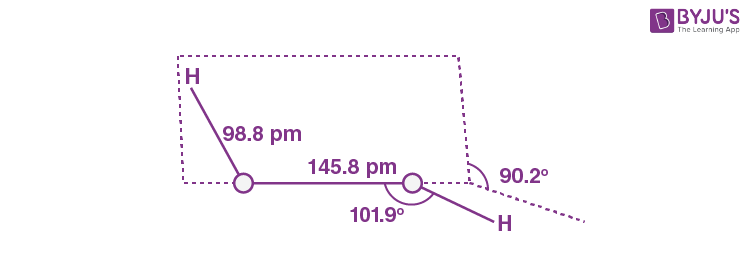Dihedral angle = 90.2°

9. The correct order of melting point is :

(A) Be > Mg > Ca > Sr

(B) Sr > Ca > Mg > Be

(C) Be > Ca > Mg > Sr

(D) Be > Ca > Sr > Mg

Sol.

Element

M.P.

Be

1560 K

Mg

924 K

Ca

1124 K

Sr

1062 K

10. The correct order of melting points of hydrides of group 16 elements is:

(A) H2S < H2Se < H2Te < H2O

(B) H2O < H2S < H2Se < H2Te

(C) H2S < H2Te < H2Se < H2O

(D) H2Se < H2S < H2Te < H2O

Sol.

Hydride

M.P.

H2O

273 K

H2S

188 K

H2Se

208 K

H2Te

222 K

11. Consider the following reaction:

A + alkali → B (Major Product)

If B is an oxoacid of phosphorus with no P-H bond, then A is:

(A) White P4

(B) Red P4

(C) P2O3

(D) H3PO3

Sol. White P4 + alkali → H3PO2

Red P4 + alkali → H4P2O6No P-H bond

12. Polar stratospheric clouds facilitate the formation of:

(A) ClONO2

(B) HOCl

(C) ClO

(D) CH4

Sol. In summer season nitrogen dioxide and methane react with chlorine monoxide and chlorine atoms forming, Chlorine sinks, preventing much ozone depletion, whereas in winter,special types of clouds called polar stratospheric clouds are formed over Antarctica. These polar stratospheric clouds provide surface on which chlorine nitrate formed gets hydrolysed to form hypochlorous acid.

$$\begin{array}{l}CI\overset{.}{O}\left ( g \right )+\overset{.}{N}O_2\left ( g \right )\rightarrow CIONO_2\left ( g \right )\end{array}$$

ClONO2(g) + H2O(g) →HOCl(g) + HNO3(g)

13. Given below are two statements:

Statement I: In ‘Lassaigne’s Test’, when both nitrogen and sulphur are present in an organic compound, sodium thiocyanate is formed.

Statement II: If both nitrogen and sulphur are present in an organic compound, then the excess of sodium used in sodium fusion will decompose the sodium thiocyanate formed to give NaCN and Na2S.

In the light of the above statements, choose the most appropriate answer from the options given below:

(A) Both Statement I and Statement II are correct.

(B) Both Statement I and Statement II are incorrect.

(C) Statement I is correct but Statement II is incorrect.

(D) Statement I is incorrect but Statement II is correct.

Sol. NaSCN + 2Na →NaCN + Na2S

14.

$$\begin{array}{l}\left ( C_7H_5O_2 \right )_2\xrightarrow[]{~~~h\nu~~~}\left [ X \right ]\rightarrow 2C_6H_5+2CO_2\end{array}$$

Consider the above reaction and identify the intermediate ‘X’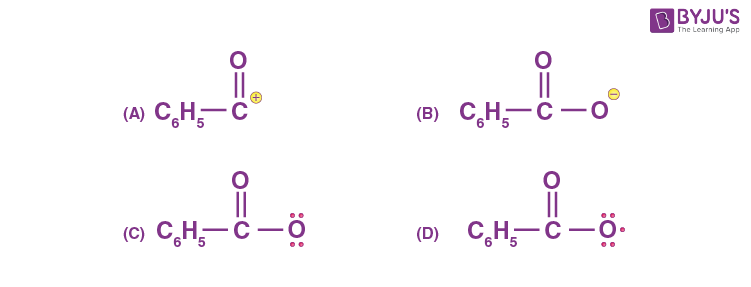Sol.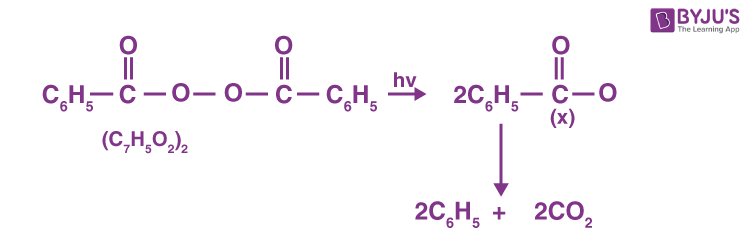15.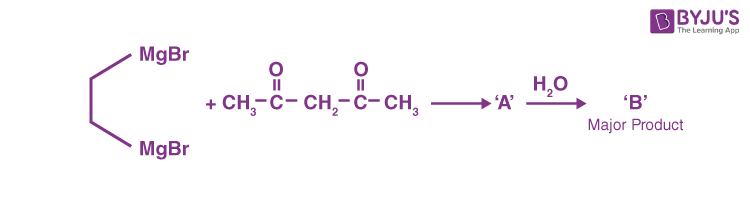Consider the above reaction sequence and identify the product B.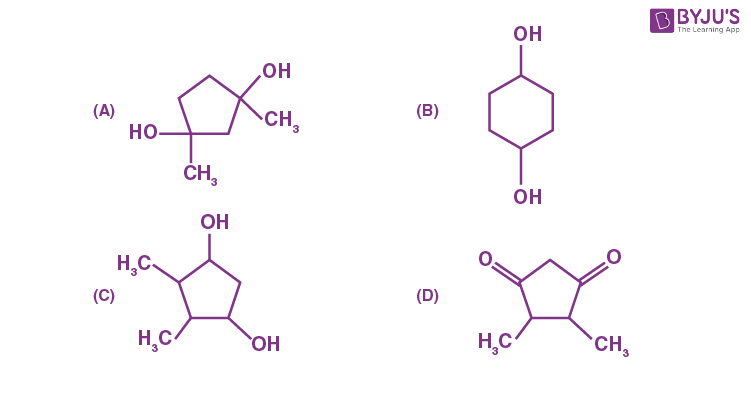Sol.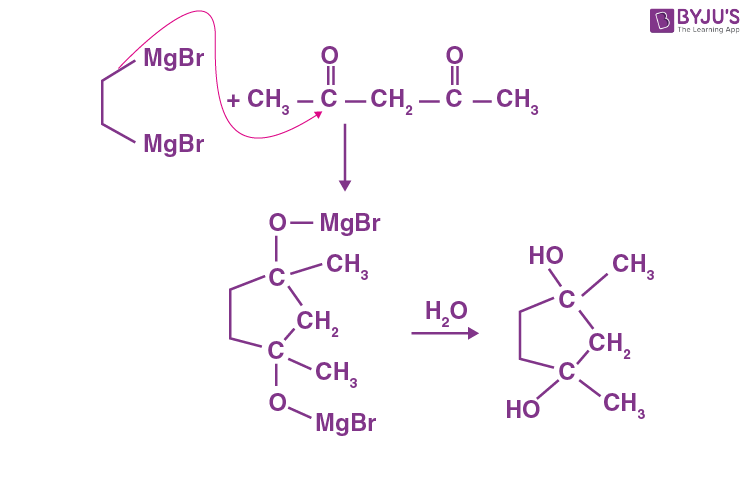16. Which will have the highest enol content?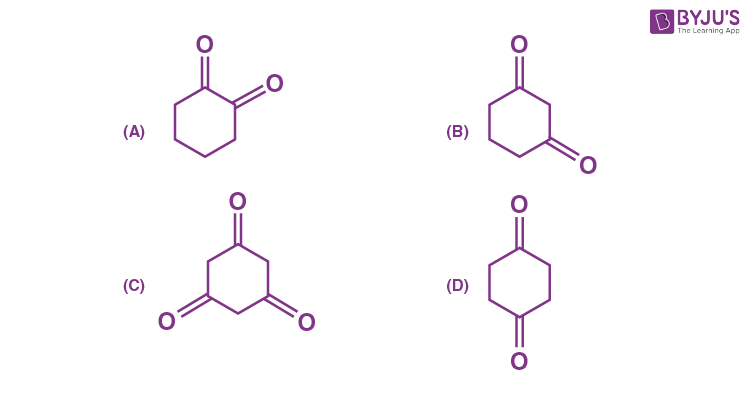Sol.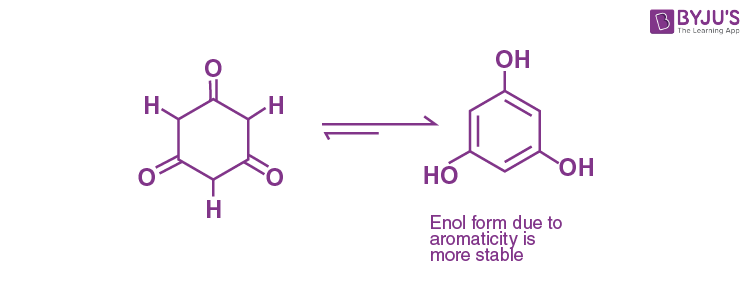17. Among the following structures, which will show the most stable enamine formation?

(Where Me is –CH3)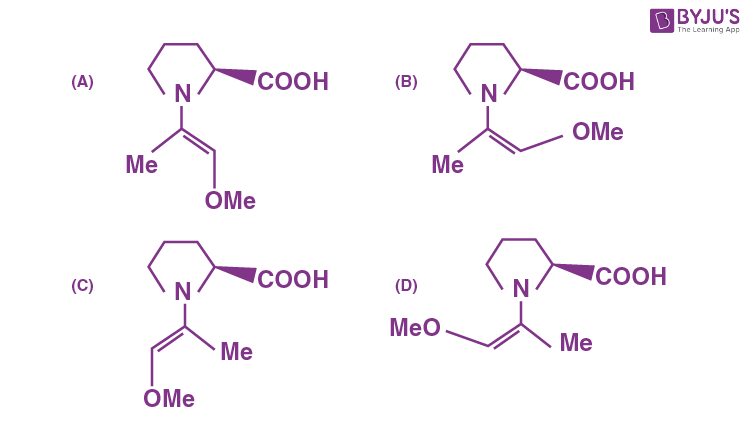Sol. The most appropriate option is (C) as one group is far enough from –COOH group.

18. Which of the following sets are correct regarding polymer?

(A) Copolymer : Buna-S

(B) Condensation polymer : Nylon-6,6

(C) Fibres : Nylon-6,6

(D) Thermosetting polymer : Terylene

(E) Homopolymer : Buna-N

Choose the correct answer from given options below:

(A) (A), (B) and (C) are correct

(B) (B), (C) and (D) are correct

(C) (A), (C) and (E) are correct

(D) (A), (B) and (D) are correct

Sol. (A) Buna-S – Copolymer

(B) Nylon-6,6 – Condensation polymer

(C) Nylon-6,6 – Fibre

(D) Terylene – Thermoplastic

(E) Buna-N – Copolymer

(A) A, B and C are correct.

19. A chemical which stimulates the secretion of pepsin is:

(A) Anti-histamine

(B) Cimetidine

(C) Histamine

(D) Zantac

Sol. Histamine stimulates the secretion of pepsin and hydrochloric acid in the stomach.20. Which statement is not true with respect to nitrate ion test?

(A) A dark brown ring is formed at the junction of two solutions.

(B) Ring is formed due to nitroferrous sulphate complex.

(C) The brown complex is [Fe(H2O)5 (NO)]SO4.

(D) Heating the nitrate salt with conc. H2SO4, light brown fumes are evolved.

Sol. Brown ring test

$$\begin{array}{l}NO_3^-+3Fe^{2+}+4H^+\rightarrow NO+3Fe^{3+}+2H_2O\end{array}$$
[Fe(H2O)6]2+ NO → [Fe(H2O)5NO]2+ H2O

Brown ring

SECTION – B

Numerical Value Type Questions: This section contains 10 questions. In Section B, attempt any five questions out of 10. The answer to each question is a NUMERICAL VALUE. For each question, enter the correct numerical value (in decimal notation, truncated/rounded-off to the second decimal place; e.g. 06.25, 07.00, –00.33, –00.30, 30.27, –27.30) using the mouse and the on-screen virtual numeric keypad in the place designated to enter the answer.

1. For complete combustion of methanol

$$\begin{array}{l}CH_3OH\left ( I \right )+\frac{3}{2}O_2\left ( g \right )\rightarrow CO_2\left ( g \right )+2H_2O\left ( I \right )\end{array}$$

the amount of heat produced as measured by bomb calorimeter is 726 kJ mol–1 at 27°C. Theenthalpy of combustion for the reaction is –x kJ mol–1, where xis ____. (Nearestinteger)

(Given : R= 8.3 JK–1 mol–1)

Sol.

$$\begin{array}{l}CH_3OH\left ( I \right )+\frac{3}{2}O_2\left ( g \right )\rightarrow CO_2\left ( g \right )+2H_2O\left ( I \right )\end{array}$$
$$\begin{array}{l}\Delta H=\Delta U+\Delta n_gRT\end{array}$$
$$\begin{array}{l}=-726~kJ+\left ( \frac{-1}{2} \right )\times8.3\times300\end{array}$$

≃ –727 kJ mol–1

2. A 0.5 percent solution of potassium chloride was found to freeze at –0.24°C. The percentagedissociation of potassium chloride is ______. (Nearest integer)

(Molal depression constant for water is 1.80 K kg mol–1 and molar mass of KCl is74.6 g mol–1)

Sol.

$$\begin{array}{l}\Delta T_f=iK_bm\end{array}$$
$$\begin{array}{l}i=\frac{0.24\times99.5\times74.6}{1.80\times0.5\times1000}\end{array}$$

= 1.98

$$\begin{array}{l}\alpha=\frac{i-1}{n-1}=\frac{0.98}{1}=0.98\end{array}$$

3. 50 mL of 0.1 M CH3COOH is being titrated against 0.1 M NaOH. When 25 mL of NaOH hasbeen added, the pH of the solution will be ____ × 10–2. (Nearest integer)

(Given : pKa (CH3COOH) = 4.76)

log 2 = 0.30

log 3 = 0.48

log 5 = 0.69

log 7 = 0.84

log 11 = 1.04

Sol. CH3COOH + NaOH → CH3COONa + H2O

at initially

$$\begin{array}{l}\underset{\textup{m~moles}}{50\times0.1}~~~~\underset{\textup{m~moles}}{25\times0.1}\end{array}$$

at time t 2.5 m moles 0 2.5 m mol

$$\begin{array}{l}pH=pK_a+log\left|\frac{\left ( \textup{salt} \right )}{\left ( \textup{acid} \right )} \right|\end{array}$$
$$\begin{array}{l}pH=4.76+log\left|\frac{2.5}{2.5} \right|\end{array}$$

pH = 4.76

4. A flask is filled with equal moles of A and B. The half lives of A and B are 100 s and 50 srespectively and are independent of the initial concentration. The time required for theconcentration of A to be four times that of B is ________s.

(Given : In 2 = 0.693)

Sol. A + B

at initially 1 1

mole mole

after 100 s

$$\begin{array}{l}\frac{1}{2}~~~~~~~~\frac{1}{4}\end{array}$$

after 200 s

$$\begin{array}{l}\frac{1}{4}~~~~~~~~\frac{1}{16}\end{array}$$

Ans. 200 seconds

5. 2.0 g of H2 gas is adsorbed on 2.5 g of platinum powder at 300 K and 1 bar pressure. Thevolume of the gas adsorbed per gram of the adsorbent is _____ mL.

(Given : R= 0.083 L bar K–1 mol–1)

Sol. PV = nRT

$$\begin{array}{l}V=\frac{2\times0.083\times300}{2\times1}=24.9~\textup{litre}\end{array}$$

$$\begin{array}{l}=\frac{24.9}{2.5}=9.96L\end{array}$$

= 9960 ml

6. The spin-only magnetic moment value of the most basic oxide of vanadium among V2O3, V2O4 and V2O5 is ______ B.M. (Nearest integer)

Sol. The most basic oxide among V2O3, V2O4 and V2O5 is V2O3

V2O3 = V+3(d2)

Magnetic moment

$$\begin{array}{l}=\sqrt{2\left ( 2+2 \right )}=\sqrt{8}\end{array}$$

= 2.83 ≈ 3

7. The spin-only magnetic moment value of an octahedral complex among CoCl3⋅4NH3, NiCl2⋅6H2O and PtCl4⋅2HCl, which upon reaction with excess of AgNO3 gives 2 moles of AgCl is _____ B.M. (Nearest Integer)

Sol.

$$\begin{array}{l}CoCl_3\cdot4NH_3\xrightarrow[\textup{excess}]{AgNO_3}\left [ Co\left ( NH_3 \right )_4\cdot Cl_2 \right ]+AgCl\end{array}$$
$$\begin{array}{l}NiCl_2\cdot6H_2O\xrightarrow[\textup{excess}]{AgNO_3}\left [ Ni\left ( H_2O \right )_6 \right ]^{2+}+2AgCl\end{array}$$
$$\begin{array}{l}PtCl_4\cdot2HCl\rightarrow\left [ PtCl_6 \right ]^{4-}+No~AgCl~ppt\end{array}$$
$$\begin{array}{l}\left [ Ni^{2+}\left( H_2O \right )_6 \right]=d^8=t\overset{6}{2}_g~\overset{2}{e}_g=2~\textup{unpaired~electrons}\end{array}$$

Magnetic moment

$$\begin{array}{l}=\sqrt{2\left ( 2+2 \right )}\end{array}$$
$$\begin{array}{l}=\sqrt{8}\approx 3\end{array}$$

8. On complete combustion 0.30 g of an organic compound gave 0.20 g of carbon dioxide and
0.10 g of water. The percentage of carbon in the given organic compound is ______.

(Nearest Integer)

Sol.

$$\begin{array}{l}C%=\frac{12}{44}\times\frac{0.20}{0.30}\times100\end{array}$$
$$\begin{array}{l}=\frac{200}{11}=18.18\approx 18\end{array}$$

9. Compound ‘P’ on nitration with dil. HNO3 yields two isomers (A) and (B) show the intramolecular and intermolecular hydrogen bonding respectively. Compound (P) on reaction with conc. HNO3 yields a yellow compound ‘C’, a strong acid. The number of oxygen atoms is present in compound ‘C’ _____.

Sol.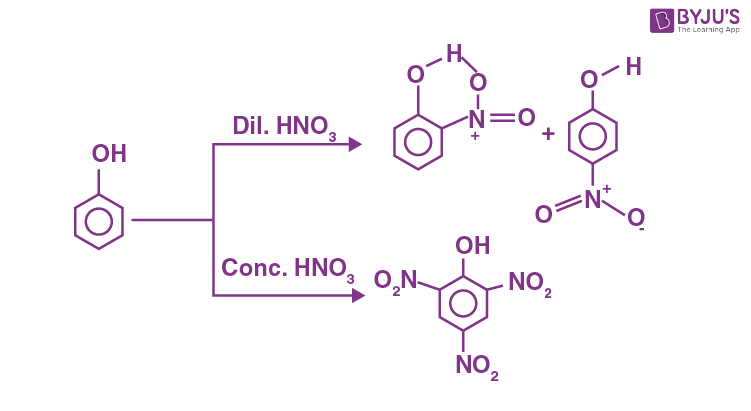The number of oxygen atoms = 7

10. The number of oxygens present in a nucleotide formed from a base, that is present only in RNA is ________.

Sol. Nucleotide formed by Uracil, the base present in RNA, is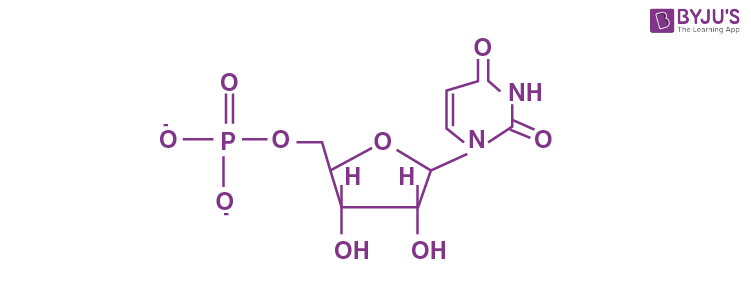The number of oxygen = 9